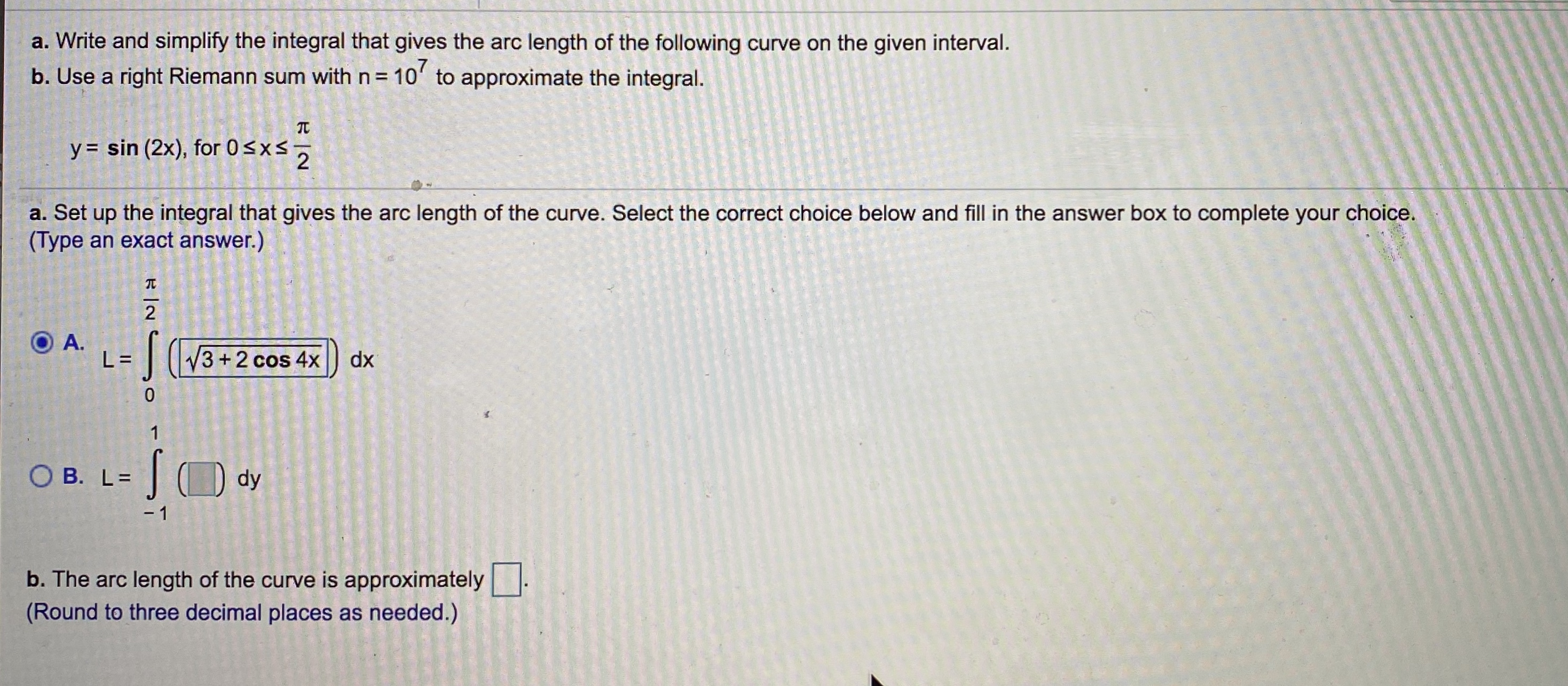# a. Write and simplify the integral that gives the arc length of the following curve on the given interval. b. Use a right Riemann sum with n= 10' to approximate the integral. y = sin (2x), for 0

Question

6.5/6.6.    4help_outlineImage Transcriptionclosea. Write and simplify the integral that gives the arc length of the following curve on the given interval. b. Use a right Riemann sum with n= 10' to approximate the integral. y = sin (2x), for 0

### Want to see this answer and more?

Experts are waiting 24/7 to provide step-by-step solutions in as fast as 30 minutes!*

*Response times may vary by subject and question complexity. Median response time is 34 minutes for paid subscribers and may be longer for promotional offers.
Tagged in
MathCalculus

### Other